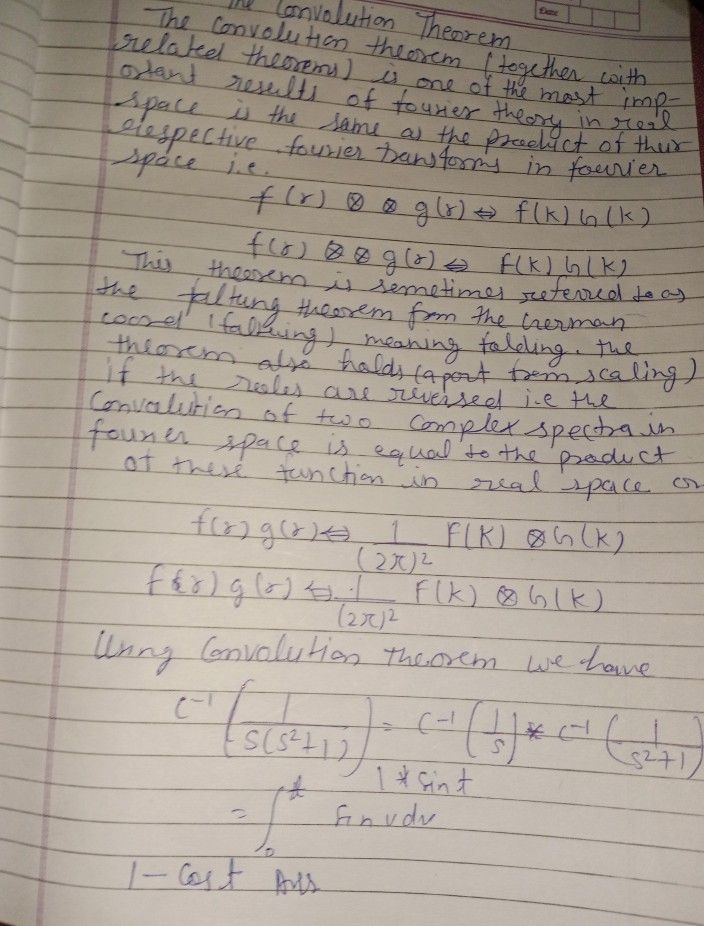Symbol
ProblemState and prove the Convolution theorem and evaluate $L^{-1}\left(\dfrac {1} {s\left(1+s^{2}\right)}\right)$ $4+3.5$
1st-6th grade
Other
Search count: 110
SolutionQanda teacher - sunil9194